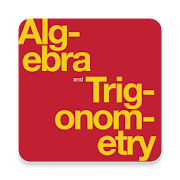# Algebra and Trigonometry Textbook & Question BankEveryone
36
Algebra and Trigonometry provides a comprehensive exploration of algebraic principles and meets scope and sequence requirements for a typical introductory algebra and trigonometry course. The modular approach and the richness of content ensures that the book meets the needs of a variety of courses. Algebra and Trigonometry offers a wealth of examples with detailed, conceptual explanations, building a strong foundation in the material before asking students to apply what they’ve learned.

1. Prerequisites
1. Introduction to prerequisites
1.1. Real numbers: algebra essentials
1.2. Exponents and scientific notation
1.4. Polynomials
1.5. Factoring polynomials
1.6. Rational expressions
2. Equations and inequalities
2. Introduction to equations and inequalities
2.1. The rectangular coordinate systems and graphs
2.2. Linear equations in one variable
2.3. Models and applications
2.4. Complex numbers
2.6. Other types of equations
2.7. Linear inequalities and absolute value inequalities
3. Functions
3. Introduction to functions
3.1. Functions and function notation
3.2. Domain and range
3.3. Rates of change and behavior of graphs
3.4. Composition of functions
3.5. Transformation of functions
3.6. Absolute value functions
3.7. Inverse functions
4. Linear functions
4. Introduction to linear functions
4.2. Modeling with linear functions
4.3. Fitting linear models to data
5. Polynomial and rational functions
5. Introduction to polynomial and rational functions
5.2. Power functions and polynomial functions
5.3. Graphs of polynomial functions
5.4. Dividing polynomials
5.5. Zeros of polynomial functions
5.6. Rational functions
5.8. Modeling using variation
6. Exponential and logarithmic functions
6. Introduction to exponential and logarithmic functions
6.1. Exponential functions
6.2. Graphs of exponential functions
6.3. Logarithmic functions
6.4. Graphs of logarithmic functions
6.5. Logarithmic properties
6.6. Exponential and logarithmic equations
6.7. Exponential and logarithmic models
6.8. Fitting exponential models to data
7. The unit circle: sine and cosine functions
7. Introduction to the unit circle: sine and cosine functions
7.1. Angles
7.2. Right triangle trigonometry
7.3. Unit circle
7.4. The other trigonometric functions
8. Periodic functions
8. Introduction to periodic functions
8.1. Graphs of the sine and cosine functions
8.2. Graphs of the other trigonometric functions
8.3. Inverse trigonometric functions
9. Trigonometric identities and equations
9. Introduction to trigonometric identities and equations
9.1. Solving trigonometric equations with identities
9.2. Sum and difference identities
9.3. Double-angle, half-angle, and reduction formulas
9.4. Sum-to-product and product-to-sum formulas
10. Further applications of trigonometry
10. Introduction to further applications of trigonometry
10.1. Non-right triangles: law of sines
10.2. Non-right triangles: law of cosines
10.3. Polar coordinates
10.4. Polar coordinates: graphs
10.5. Polar form of complex numbers
10.6. Parametric equations
10.7. Parametric equations: graphs
10.8. Vectors
11. Systems of equations and inequalities
11. Introduction to systems of equations and inequalities
11.1. Systems of linear equations: two variables
11.2. Systems of linear equations: three variables
11.3. Systems of nonlinear equations and inequalities: two variables
11.4. Partial fractions
11.5. Matrices and matrix operations
11.6. Solving systems with gaussian elimination
11.7. Solving systems with inverses
11.8. Solving systems with cramer's rule
12. Analytic geometry
12. Introduction to analytic geometry
12.1. The ellipse
12.2. The hyperbola
12.3. The parabola
12.4. Rotation of axes
12.5. Conic sections in polar coordinates
13. Sequences, probability, and counting theory
Collapse

## Reviews

Review Policy
4.3
36 total
5
4
3
2
1

Updated
March 20, 2018
Size
6.6M
Installs
10,000+
Current Version
2.1.1
Requires Android
4.4 and up
Content Rating
Everyone
Interactive Elements
Users Interact
Permissions
Offered By
QuizOver.com